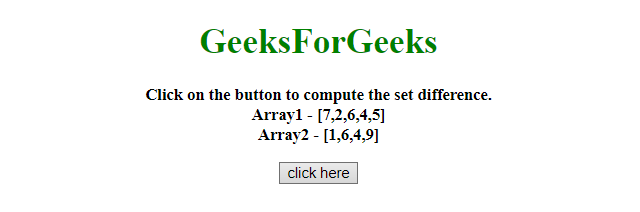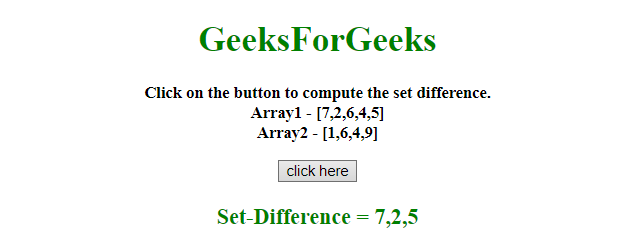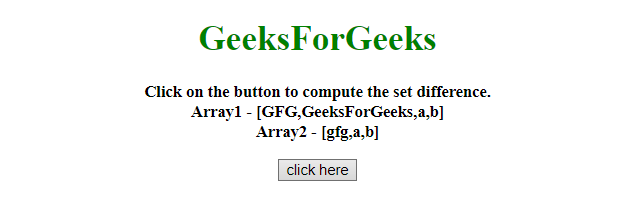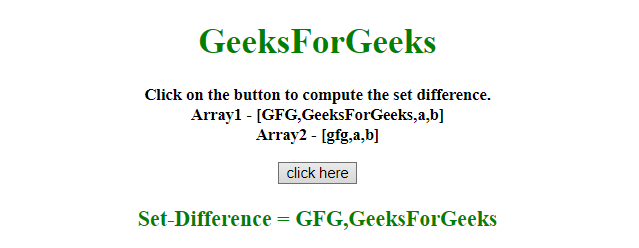# Compute the set difference using JavaScript arrays

Given a two array and the task is to compute the set difference between the two Arrays using JavaScript.

Approach:

• Store the both array values into the two variables.
• Use filter() method for every value of array_1, if there is a value in array_2 then not include it. Otherwise include the value of array_1.

Example 1: In this example, the set difference is calculated using the filter() method.

 `  ` `<``html``>  ` ` `  `<``head``>  ` `    ``<``title``>  ` `        ``Compute the set difference using ` `        ``Javascript arrays ` `    `` ` ` ` ` `  `<``body` `style` `= ``"text-align:center;"``>  ` `     `  `    ``<``h1` `style` `= ``"color:green;"` `id` `= ``"h1"``>  ` `        ``GeeksForGeeks  ` `    `` ` `     `  `    ``<``p` `id` `= ``"GFG_UP"` `style` `=  ` `        ``"font-size: 15px; font-weight: bold;"``> ` `    `` ` `     `  `    ``<``button` `onclick` `= ``"GFG_Fun()"``> ` `        ``click here ` `    `` ` `     `  `    ``<``p` `id` `= ``"GFG_DOWN"` `style` `=  ` `        ``"color:green; font-size: 20px; font-weight: bold;"``> ` `    `` ` `     `  `    ``<``script``>  ` `        ``var up = document.getElementById('GFG_UP'); ` `        ``var down = document.getElementById('GFG_DOWN'); ` `        ``var A = [7, 2, 6, 4, 5]; ` `        ``var B = [1, 6, 4, 9]; ` `        ``up.innerHTML = "Click on the button to compute " ` `                ``+ "the set difference.<``br``>" + "Array1 - [" ` `                ``+ A + "]<``br``>Array2 - [" + B + "]"; ` `         `  `        ``function GFG_Fun() { ` `            ``diff = A.filter(x => !B.includes(x) ) ` `            ``down.innerHTML = "Set-Difference = " + diff; ` `        ``} ` `    ``  ` `  ` ` `  `     `

Output:

• Before clicking on the button:• After clicking on the button:Example 2: In this example, the set difference is calculated using the filter() method but by a bit different approach.

 `  ` `<``html``>  ` ` `  `<``head``>  ` `    ``<``title``>  ` `        ``Compute the set difference ` `        ``using Javascript arrays ` `    `` ` ` ` ` `  `<``body` `style` `= ``"text-align:center;"``>  ` `     `  `    ``<``h1` `style` `= ``"color:green;"` `id` `= ``"h1"``>  ` `        ``GeeksForGeeks  ` `    `` ` `     `  `    ``<``p` `id` `= ``"GFG_UP"` `style` `=  ` `        ``"font-size: 15px; font-weight: bold;"``> ` `    `` ` `     `  `    ``<``button` `onclick` `= ``"GFG_Fun()"``> ` `        ``click here ` `    `` ` `     `  `    ``<``p` `id` `= ``"GFG_DOWN"` `style` `= ` `        ``"color:green; font-size: 20px; font-weight: bold;"``> ` `    `` ` `     `  `    ``<``script``>  ` `        ``var up = document.getElementById('GFG_UP'); ` `        ``var down = document.getElementById('GFG_DOWN'); ` `        ``var A = ["GFG", "GeeksForGeeks", "a", "b"]; ` `        ``var B = ["gfg", "a", "b"]; ` `         `  `        ``up.innerHTML = "Click on the button to compute" ` `                ``+ " the set difference.<``br``>" + "Array1 - [" ` `                ``+ A + "]<``br``>Array2 - [" + B + "]"; ` `         `  `        ``function GFG_Fun() { ` `            ``diff = A.filter(function(x) { ` `                ``return B.indexOf(x) < ``0`  `            ``}) ` `             `  `            ``down.innerHTML` `= ``"Set-Difference = "` `+ diff; ` `        ``} ` `    ``  ` `  ` ` `  `                     `

Output:

• Before clicking on the button:• After clicking on the button:My Personal Notes arrow_drop_upCheck out this Author's contributed articles.

If you like GeeksforGeeks and would like to contribute, you can also write an article using contribute.geeksforgeeks.org or mail your article to contribute@geeksforgeeks.org. See your article appearing on the GeeksforGeeks main page and help other Geeks.

Please Improve this article if you find anything incorrect by clicking on the "Improve Article" button below.